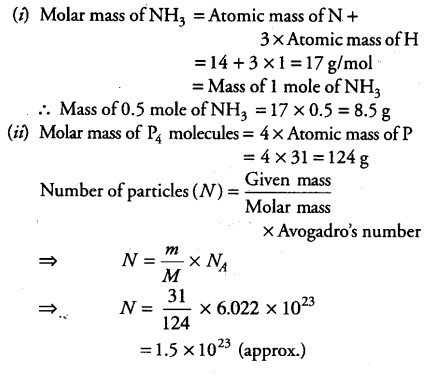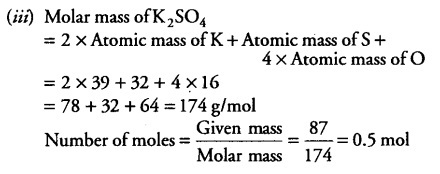# What is the mass of 0.5 mole of NH3?

(i) what is the mass of 0.5 mole of NH3? Given atomic mass of N = 14 u, atomic mass of H =1 u.
(ii) Calculate the number of particles in 31 g of P4 molecules. (Atomic mass of P =31u)
(iii) Find the number of moles in 87 g of K2S04.
(Atomic masses of K = 39 u, S = 32 u andO= 16 u)